You often have a set of questions where you wish to total the answers. For example:

• asking how many hours a week respondents spend doing different things
• asking how much money respondents spent on different activities during a visit
• asking respondents to share a fixed amount or percentage between a set of options

The amount allocated to each option gives the relative importance of each. This gives good numeric data for analysis, but is quite difficult for respondents.

This worksheet shows you how to do this using the Totalize (or Totalise) function in the Snap Toolkit. The Totalize function calculates a running total as the respondent completes the answers. It explains how to create a set of Open First and Open Next questions for quantity responses, and use Totalize to put a running total into the final response. It also shows you how to set a valid range on the total, so that respondents cannot move to the next page if the total has overrun.

### Background

The Totalize function works on a group of questions in Snap consisting of the options to be added up and the running total. The running total can be anywhere in the group. The questions must be sequential and on a single web page. You can have several Totalize groups in a single survey.

You run Totalize after you have published your survey. It inserts extra code in the published survey that displays the running total. The total is calculated according to your settings in Totalize.

You can set a limit on the running total in Snap using the Valid option in Variable properties for that question (for example, setting a valid range of 0 to 100). If you have set a limit on the total, the answers are checked against the valid range when the user goes to the next page. If they fall outside it, a message is displayed. You can customize the default message text, see the section “Changing specific texts” in the help topic: Hiding question numbers in web surveys and changing associated text.

### Step 1: Create your set of questions

This worksheet uses a new survey with a single set of questions to totalize. The first step is to create the group of questions that need to be totalled. These must be quantity questions.

1. Create your web or Snap WebHost survey.
2. Select Open First as the style of the first question and Quantity as the Response type.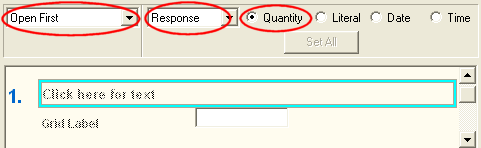3. Enter the question text, for example “How did you spend your day at the activity centre? Please allocate hours to the activities so the total is 8 or fewer.
4. Enter the first activity as the grid label.
5. Press [Tab] to create the next question in the list. By default, Snap will create this as an Open Next question with a Quantity response. Enter an activity as the grid label. Repeat until you have entered all your activities and add an extra grid question to display the running total.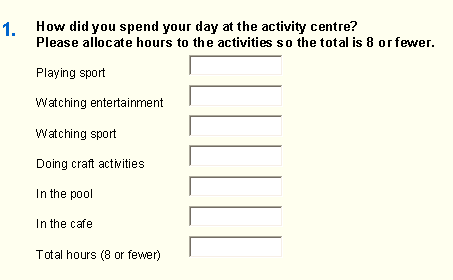6. For this example, place a valid range on the Total question to ensure that the total number of hours does not exceed eight. (It would normally be adequate to sum the hours, as this makes it more complicated for the respondent. Doing it this way is purely as a demonstration.) Select the Total question and press [Alt] + [Enter] to open the Variable Properties window.
7. Scroll down to the Valid entry and enter 0 to 8.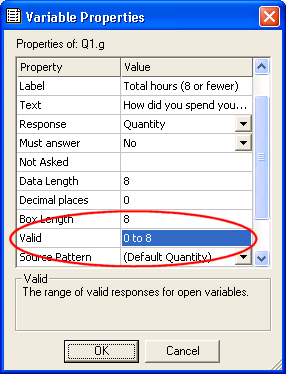8. Click [OK].
9. Select File|Publishto publish the survey. Make a note of where the published survey will be stored (the path).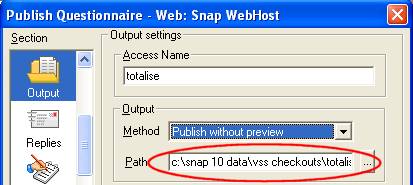### Step 2: Totalize the set of questions

1. Open the Totalize program. You would normally do this by Selecting [Start] on the Microsoft toolbar, then type Totalize (this will be Totalise if you are in the UK) in the ‘Search programs and files’ box.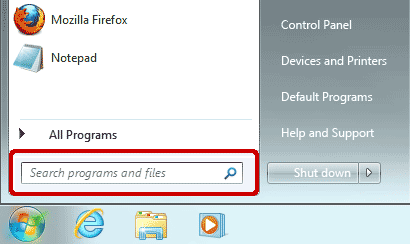2. Click the [Browse] button and select the published survey file.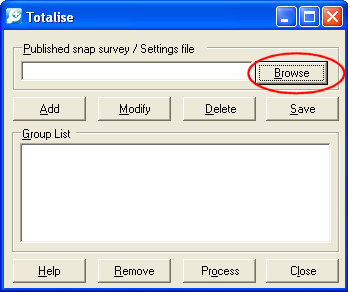3. Click [Add] to define the group of questions to total.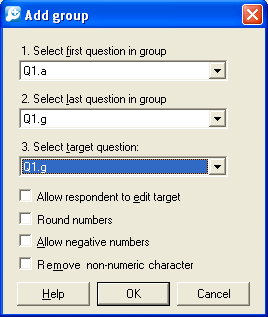4. Select the first question in the survey that contributes to the total.
5. Select the last question in the survey that contributes to the total or is the total question. (The total is usually the last question in the group, but you could have a layout in which it is not the last question.)
6. Select the question that displays the total as the target question.
7. Click [OK] to define the totalize group. A summary of the group details appears in the Totalize window.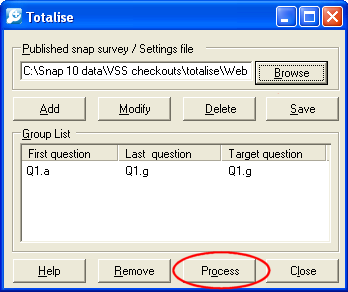8. Click [Process] to add the running total code to the published survey.
9. Run your survey as normal. When respondents complete a question in the group, their answer will be added to the total.If you re-publish the survey, the totalize information will be lost. You will have to add it again. You can do this quickly by clicking [Save] in Totalize to save your settings and then browsing to the settings file to add the settings back to the survey. This only works if you have not changed the question variables in the survey.

### Conclusion

This worksheet has explained how to use the Totalize function to add a running total to a group of questions in a published survey. It also describes how to set a valid range on the total figure to ensure that responses do not exceed a permitted maximum.

If you do not need to display a running total, you can use derived variables to calculate totals. Examples of calculating values using a derived variable are available in the worksheet: Calculating the difference between times on different dates and the help page: Calculating age from a date of birth. There is also reference information about derived variables.

If you are entering data using the Data Entry screen within Snap you can also display running totals by including calculations in the questionnaire.

For more information about setting valid ranges on questions, see the section: Setting valid ranges on a question.

There is help available for the Totalize tool if you click the [Help] button on the tool windows.

If there is a topic you would like a worksheet on, email to snapideas@snapsurveys.com